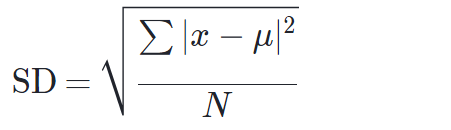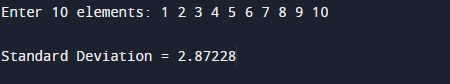# C++ Program to Calculate Standard Deviation

In this tutorial you will learn about the C++ Program to Calculate Standard Deviation and its application with practical example.

In this tutorial, we will learn to create a  c++  Program to Calculate Standard Deviation.

## Prerequisites

Before starting with this tutorial we assume that you are best aware of the following C programming topics:

• C++ Operators.
• Functions.
• For Loop and while loop.
• Array.
• String.

## What Is Standard Deviation.?

The concept behind Standard Deviation is a measure of how spread out numbers are in a given Scenario.In Mathematical  term, the Standard Deviation is  measure of the amount of variation on set of values.

formula is Standard deviation  is :=>where ∑ sum means “sum of”.
x is a value in the data set.
μ is the mean of the data set.
and N is the number of data points.

in simple form Standard deviation is .

Find the mean ∑.
Find variation (Variance, take each difference, square it, and then average the result)
Sum the values from above step and divide by total numbers.
Standard Deviation is just the square root of Variance.

## C++ Program to Calculate Standard Deviation

In this program , we will calculate Standard Deviation of a given numbers .First of all user will be prompted to enter a number and afterword passing these value to function to calculate Standard deviation.

## OutputIn the above program, we have first declared and initialized a set variables required in the program.

• arr[] = it will hold entered elements.
• value = it will hold a elements values.
• i=  for iteration.
• sum= add all elements to find mean.
• mean=hold the mean value.
• sd= gives us standard deviation.

Now let us look method to find standard deviation step by step.

1. first or all take elements from user.
2. then pass these elements to function SD.
3. where first we calculate the mean (average) of each data set.
4. then,subtracting the mean from each number.
5. then,Square each deviation.
6. after that add all the squared deviations.
7. Divide the value obtained  by the number of items in the data set.
8. Calculate the square root of the value obtained (Variance).
9. And at the return the sd to main function were we print standard deviation.

In this tutorial we have learn about the C++ Program to Calculate Standard Deviation and its application with practical example. I hope you will like this tutorial.